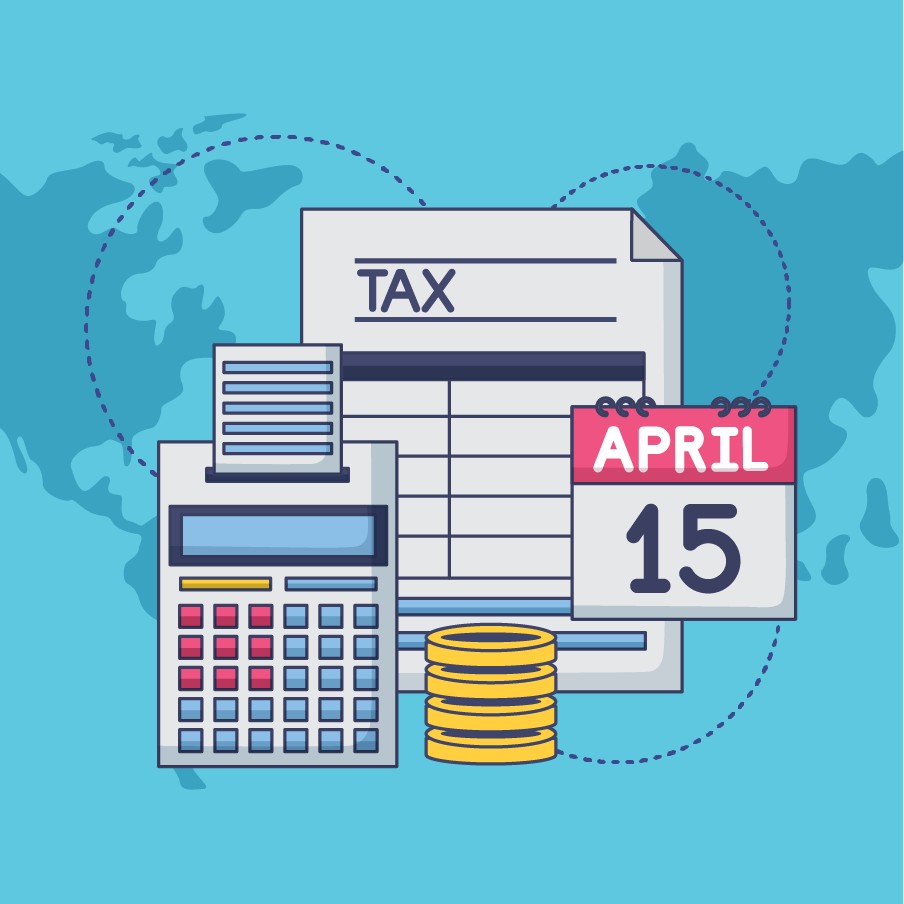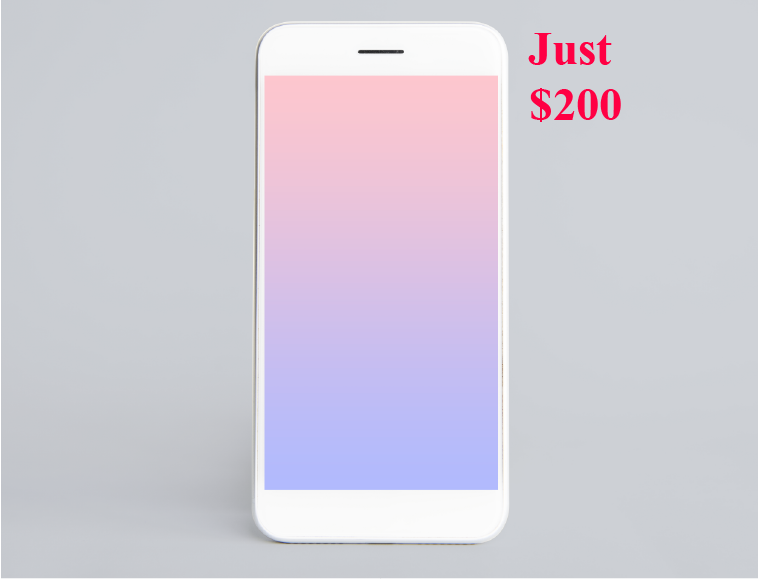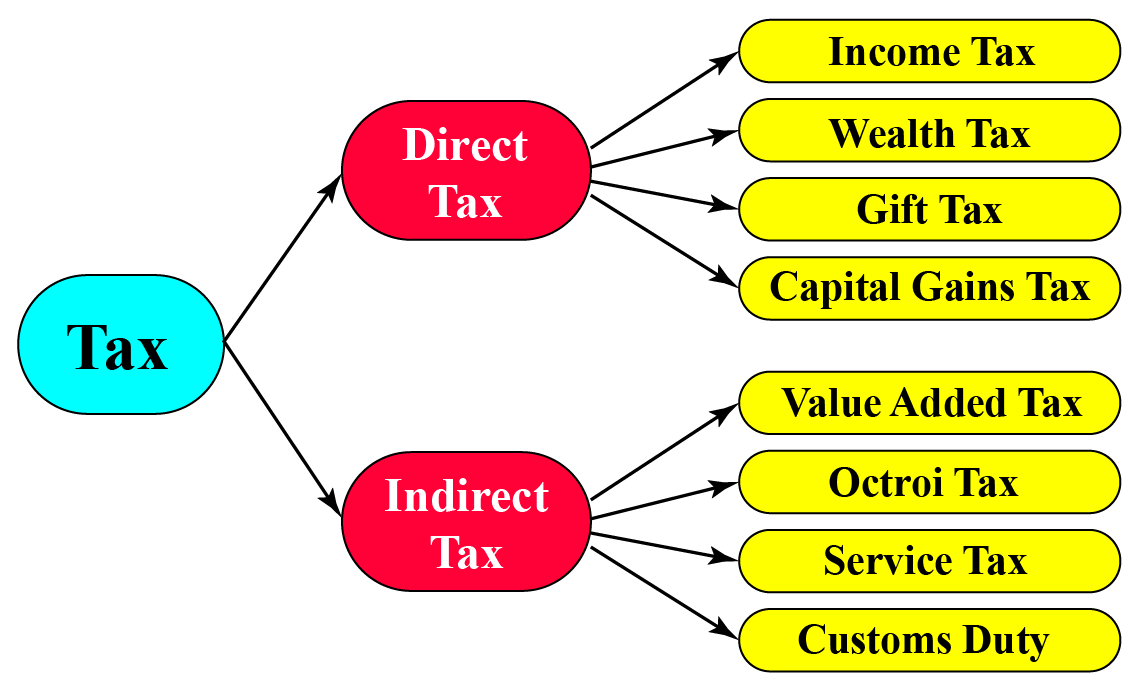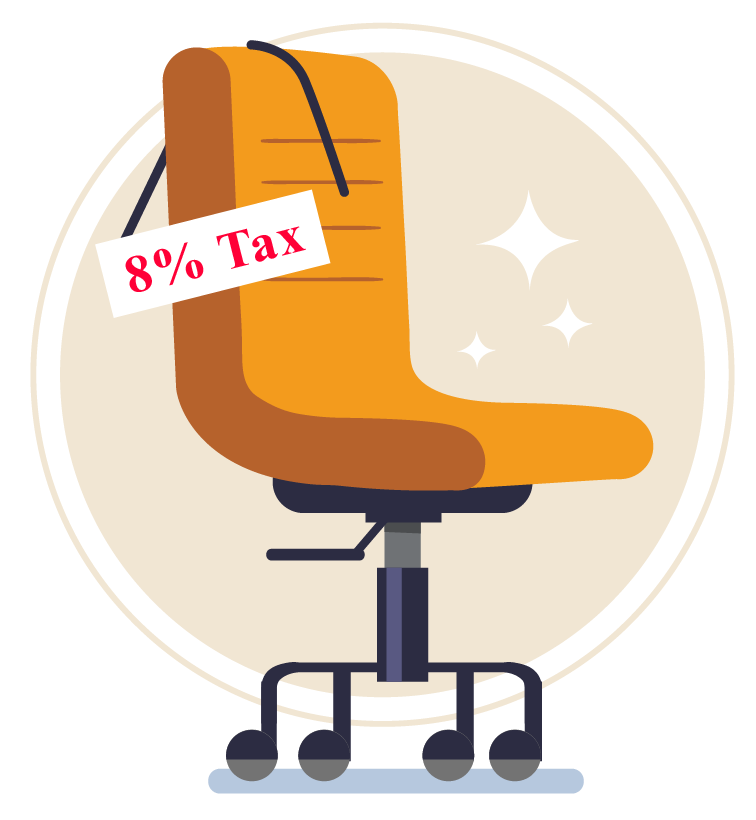# Taxes

Emma once heard her mother discussing about the payment of income tax.

She was curious to know more about taxes.In this mini-lesson, we will learn about taxes including its definition and types with the help of some solved examples and a few interactive questions for you to test your understanding.

## Lesson Plan

 1 What Are Taxes? 2 Important Notes on Taxes 3 Solved Examples on Taxes 4 Challenging Questions on Taxes 5 Interactive Questions on Taxes

## What Are Taxes?

The amount paid by the people to the government for goods and services provided is called tax.

There are two parties involved in any tax transaction. They include a tax payer and a tax collector.

A tax payer is the person or an organization who is paying the tax to the government and a tax collector is the government or any middleman collecting tax on behalf of the government.

You may have heard terms like income tax, value added tax, service tax, etc. The government charges a fee on every item that is sold. This fee is meant to be spent on public welfare and is known as tax. The tax charged is always a percentage of the selling price.

The formula used to calculate tax on the selling price is given below:

 Tax amount = $$(S.P. \times \dfrac{Tax\ rate}{100})$$

Let's consider an example.

Let’s say an item costs $$50$$ and a sales tax of $$5 \%$$ was charged. What would be the bill amount?

Let’s first find $$5 \%$$ of $$50.$$

\begin{align}{5 \over 100} \times 50 = 2.5 \end{align}

Now let’s add this amount to the cost price.

\begin{align}50 + 2.5 = 52.5 \end{align}

The total bill amount including taxes would be $$52.5$$

When the tax amount and the selling price (S.P.) of an item are given and we have to calculate the tax rate, then we can use the following formula:

 Tax rate = $$\dfrac{Tax\ amount}{Price\ before\ tax}\times 100\%$$

Consider the following example.

You purchase a mobile phone whose price before tax is $200 and the tax paid on it is$20.Then, the rate of tax paid on that mobile can be calculated by using the above formula.

$Tax\ rate=\dfrac{Tax\ amount}{Price\ before\ tax}\times 100%$

$Tax\ rate=\dfrac{20}{200}\times 100%$

$Tax\ rate=10\%$

Let’s now try and understand how taxes are levied on a discounted product.

-  Discount is calculated on the selling price excluding taxes.
-  The tax is applied on the amount arrived at after subtracting the discount value from the selling price.

Consider the following scenario.

You have ordered a pizza at a restaurant and it costs $$12.$$The restaurant is offering a discount of $$30 \%$$ on all dine-in orders.

Hence, the final amount, assuming the restaurant only charges $$10 \%$$ service tax, is calculated in the following way.

First, calculate the discounted price.

\begin{align} \text{Discount} & = {30 \over 100} \times 12\\ & = 3.6 \end{align}

\begin{align} \text{Final Bill Amount} & =12-3.6 \\ & =8.4 \end{align}

Remember, the tax is charged on the discounted price. We know that $$10 \%$$ service tax was charged on the final amount.

\begin{align} {10 \over 100} \times 8.4 = 0.84 \end{align}

\begin{align} \text{Final Amount} &= 8.4 + 0.84 \\ & = 9.24 \end{align}

## Different Types of Taxes

There are two types of taxes.

They are direct and indirect taxes.Taxes paid by the citizens directly to the government are direct taxes.

For example, income tax, corporate tax, etc. are categorized as direct taxes.

Taxes paid by the people but not directly collected by the government are indirect taxes.

For example, sales tax, entertainment tax, excise duty, etc. are categorized as indirect taxes.

Indirect taxes are collected by the people who sell goods or services. These include shop owners and businessmen, who then further pay the indirect tax to the government.

Indirect tax can be passed from one entity to another.

## Tax on Marked Price

We calculate tax on the marked price when the seller sells an item at the marked price without any discount.

In that case, the marked price and the selling price are the same. Thus, tax is calculated on the marked price.

For example, if the marked price on a chair is $1200 and 8% tax is to be paid to the shopkeeper, then the amount of tax to be paid to the shopkeeper can be calculated as:Tax amount = $$(M.P. \times \dfrac{Tax\ rate}{100})$$ = $$(1200 \times \dfrac{8}{100})$$ = $$(12 \times 8)$$ = $$96$$ $$\therefore$$ We need to pay$96 as tax on the purchase of the chair of marked price \$1200.

Always remember that only when the final selling price is the same as the marked price of the item purchased, we calculate tax on the marked price.

Otherwise, we calculate tax on the net amount that the customer is paying to buy a product or a service.

More Important Topics
Numbers
Algebra
Geometry
Measurement
Money
Data
Trigonometry
Calculus
More Important Topics
Numbers
Algebra
Geometry
Measurement
Money
Data
Trigonometry
Calculus
Learn from the best math teachers and top your exams

• Live one on one classroom and doubt clearing
• Practice worksheets in and after class for conceptual clarity
• Personalized curriculum to keep up with school